Courses

# Bond Stress Civil Engineering (CE) Notes | EduRev

## Civil Engineering (CE) : Bond Stress Civil Engineering (CE) Notes | EduRev

The document Bond Stress Civil Engineering (CE) Notes | EduRev is a part of the Civil Engineering (CE) Course Civil Engineering SSC JE (Technical).
All you need of Civil Engineering (CE) at this link: Civil Engineering (CE)

Chapter 4

Bond Stress

Bond, Anchorage, Development Length and Torsion One of the most important assumptions in the behaviour of reinforced concrete structures is that there is proper bond between concrete and reinforcement bars. When steel bars are embedded in concrete, the concrete, after setting, adheres to the surface of the bars and thus resists any force that tends to pull 0r push this bond. The intensity of this adhesive force is called bond stress. Thus the term 'bond' is used to describe the means by which slip between steel and concrete is prvented. The bond stresses are the longitudinal shearing stresses acting on the surface between the steel and concrete.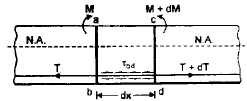Figure shows two sections ab and cd, spaced dx apart of a reinforced concrete beam. Let M be the bending moment at section ab and M + dM be the bending moment at section cd.

Similarly let T and T + dT be the tensile forces developed in steel reinforcement at ab and cd respectively.
Now, M = T. jd and M + dM = (T+dT)d Hence, dM = dT. jd or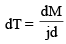The difference in the tensile force (dT) in steel bars between sections is resisted by bond stress developed in the length dx,.
Let, ζ bd = bond stress developed in concrete around the steel reinforcement.

∑0 = sum of the perimeters of the steel bars resisting tension.
Total surface area of bars = d× ∑0
Hence, ζ bd [d × ∑0 ]        ...(ii)

Substituting the value of dT in (i), we get,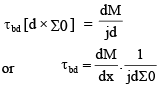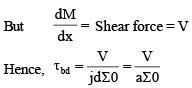The stress ζ bd at a particular sections is called bond stress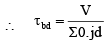where V = Shear force at any section
d = Effective depth of the section
∑0 = Sum of all perimeter of reinforcement
= n. p (φ)

where n = Number of reinforcment and φ = diamter of reinforcement

Permissible Bond Stress
As per IS 456 : 2000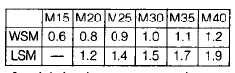These value of bond stress is for plain bar in tension For deformed bar the above value should be increased by 60%.
For bar in compression the above value should be increased by 25%.

Development Length (Ld)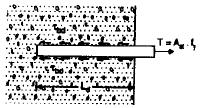Figure shows a steel bar embedded in concrete and subjected to a tensile force T. Due to this force, there will be tendency of the bar to slip out and this tendency is resisterd by the bond stress is developed along the length. The required length, necessary to develop full resisting force, is called anchorage length in case of axial tension (or compression) and development length in the case of flexural tension, and is designated by symbol Ld.
Hence, if φ is the nominal diameter of the bar, we have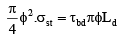or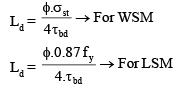Checking Development Lengths of Tension Bars

The computed stress ( σs ) in a reinforcement bar, at every section must be developed on both the sides of the section. This is done by providing development length Ld to both the sides of the section. Such a development length is usually available at mid-span location where positive (or sagging) BM is maximum for simply supported beams. Similarly, such a development is usually available at the intermediate support of a continuous beam where negative (or hogging) BM is maximum. Hence no special checking may be necessary in such locations. However special checking for development lengths is essential at the following:

1. At simple supports
2. At cantilever supports
3. In flexural members that have relatively short spans
4. At point of contraflexure.
5. At lap splices
6. At point of bar cutoff
7. For stirrups and transverse ties

Torsion

Equivalent shear force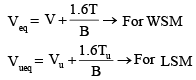•  Nominal shear stress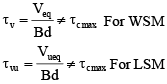Equivalent moment

M = meq + mueq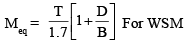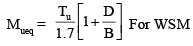Transverse reinforcement

As per IS : 456–2000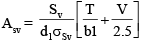Also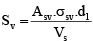where,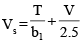Here, T = Torsional moment
Sv = Spacing the stirrup rienforcement
b1 = Centre to centre distance between cor ner bars in the direction of width
d1 = Centre to centre distance between cor ner bars in the direction of depth
σsv = Permissible tensile stress in shear re  inforcement .

Maximum spacing for Transverse reinforcement
(i)X1
(ii)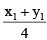(iii) 300 mm When a beam is subjected to torsion, if depth of the beam is more than 450 mm or for a beam not subjected to torsion if depth of web exceeds 750 mm, then side face reinforcement equal to 0.1% of crosssectional area is equally distributed on both faces of the beam.

Offer running on EduRev: Apply code STAYHOME200 to get INR 200 off on our premium plan EduRev Infinity!

## Civil Engineering SSC JE (Technical)

109 docs|50 tests

,

,

,

,

,

,

,

,

,

,

,

,

,

,

,

,

,

,

,

,

,

;